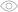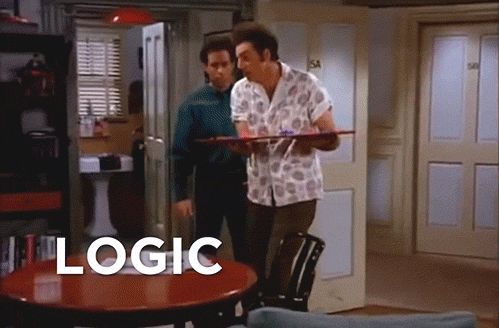# Minimal Government and Maximum Freedom: Test Your Understanding with the Libertarianism Quiz365Are you familiar with the basics of logic and reasoning? This quiz on logic will test your knowledge of the fundamental principles and methods of logical argumentation. From syllogisms to propositional logic to the foundations of mathematical reasoning, this quiz will challenge and educate you on one of the most fundamental and widely studied branches of philosophy.

What is the contrapositive of a proposition If P, then Q?

If P, then not Q

If not P, then Q

If Q, then not P

If not Q, then not P

Which of the following is NOT a valid argument form?

Modus Ponens

Modus Tollens

Affirming the Consequent

Denying the Antecedent

What is the Law of Excluded Middle?

A proposition is either true or false

A proposition cannot be both true and false at the same time

A proposition must be either true or false

A proposition must be either false or not true

What is the concept of Proof by Contradiction?

Proving a proposition by assuming its negation and deriving a contradiction

Proving a proposition by assuming it is false and deriving a contradiction

Proving a proposition by assuming its negation and deriving a proof

Proving a proposition by assuming it is false and deriving a proof

What does the term sound argument mean in logic?

A valid argument with true premises

A valid argument with false premises

An invalid argument with true premises

An invalid argument with false premises

What is the difference between Necessary and Sufficient conditions?

Necessary conditions are those that must be present for a proposition to be true, while sufficient conditions are those that are enough for a proposition to be true

Sufficient conditions are those that must be present for a proposition to be true, while necessary conditions are those that are enough for a proposition to be true

Necessary conditions are those that are enough for a proposition to be true, while sufficient conditions are those that must be present for a proposition to be true

Sufficient conditions are those that are enough for a proposition to be false, while necessary conditions are those that must be present for a proposition to be false

What is the concept of Syllogism?

A form of argument in which two premises lead to a conclusion

A form of argument in which one premise leads to a conclusion

A form of argument in which three premises lead to a conclusion

A form of argument in which four premises lead to a conclusion

What is the difference between Inductive Reasoning and Deductive Reasoning?

Inductive Reasoning involves inferring a general principle from specific examples, while Deductive Reasoning involves deriving a specific conclusion from general principles

Deductive Reasoning involves inferring a general principle from specific examples, while Inductive Reasoning involves deriving a specific conclusion from general principles

Inductive Reasoning involves deriving a specific conclusion from general principles, while Deductive Reasoning involves inferring a general principle from specific examples

Deductive Reasoning involves deriving a general principle from specific examples, while Inductive Reasoning involves inferring a specific conclusion from general principles

What is a Premise?

A conclusion in an argument

A reason for a conclusion in an argument

A fact in an argument

An assumption in an argument

Very impressive!

Well done on your outstanding score in the Logic quiz! Your grasp of the principles and techniques of logical reasoning reflects your dedication to critical thinking and problem solving. Keep up the great work!

There is still room for improvement!

It's okay if you didn't do as well as you hoped on this quiz. It's important to remember that everyone has room for improvement and it's a great opportunity to learn something new. Take some time to study the material and try again. With practice and dedication, you'll be able to do better next time. Don't give up!

What is the contrapositive of a proposition If P, then Q?
1 / 9Which of the following is NOT a valid argument form?
2 / 9What is the Law of Excluded Middle?
3 / 9What is the concept of Proof by Contradiction?
4 / 9What does the term sound argument mean in logic?
5 / 9What is the difference between Necessary and Sufficient conditions?
6 / 9What is the concept of Syllogism?
7 / 9What is the difference between Inductive Reasoning and Deductive Reasoning?
8 / 9What is a Premise?
9 / 9### Calculating results...

Useful and short ads help us create new content every day.